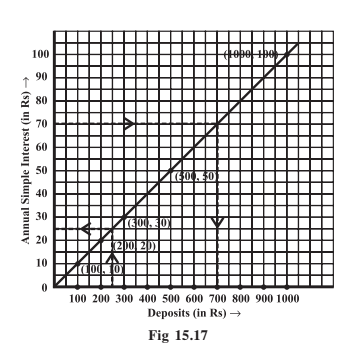# Q. A bank gives 10% Simple Interest (S.I.) on deposits by senior citizens. Draw a graph to illustrate the relation between the sum deposited and simple interest earned. Find from your graphTry a case of direct variation?

Taking a case of direct variation the graph is as shown below.

Taking sum deposite as 100,200,300,500 ,1000.

and  10% Simple Interest (S.I.).Exams
Articles
Questions